# Linear Modeling in Python¶

This notebook, which was created and presented by Skipper Sebold at the Scipy2012, introduces the use of pandas and the formula framework in statsmodels in the context of linear modeling.

It is based heavily on Jonathan Taylor's class notes that use R

In :
import matplotlib.pyplot as plt
import pandas
import numpy as np

from statsmodels.formula.api import ols
from statsmodels.graphics.api import interaction_plot, abline_plot, qqplot
from statsmodels.stats.api import anova_lm

In :
%matplotlib inline


## Example 1: IT salary data¶

In this example we will first establish a linear model, of salary as a function of experience, education, and management level. We will test for any interactions between these factors. Significant interactions will be included in the model. Finally, we will remove outliers, and plot the resulting fits.

### Get the data¶

Outcome: S, salaries for IT staff in a corporation Predictors: X, experience in years M, managment, 2 levels, 0=non-management, 1=management E, education, 3 levels, 1=Bachelor's, 2=Master's, 3=Ph.D
In :
url = 'http://stats191.stanford.edu/data/salary.table'
salary_table = pandas.read_table(url) # needs pandas 0.7.3
#salary_table.to_csv('salary.table', index=False)


### Inspect the data¶

In :
print(salary_table.head(10))

       S  X  E  M
0  13876  1  1  1
1  11608  1  3  0
2  18701  1  3  1
3  11283  1  2  0
4  11767  1  3  0
5  20872  2  2  1
6  11772  2  2  0
7  10535  2  1  0
8  12195  2  3  0
9  12313  3  2  0

In :
E = salary_table.E # Education
M = salary_table.M # Management
X = salary_table.X # Experience
S = salary_table.S # Salary


Let's explore the data

In :
fig = plt.figure(figsize=(10,8))
xlim=(0, 27), ylim=(9600, 28800))
symbols = ['D', '^']
man_label = ["Non-Mgmt", "Mgmt"]
educ_label = ["Bachelors", "Masters", "PhD"]
colors = ['r', 'g', 'blue']
factor_groups = salary_table.groupby(['E','M'])
for values, group in factor_groups:
i,j = values
label = "%s - %s" % (man_label[j], educ_label[i-1])
ax.scatter(group['X'], group['S'], marker=symbols[j], color=colors[i-1],
s=350, label=label)
ax.legend(scatterpoints=1, markerscale=.7, labelspacing=1);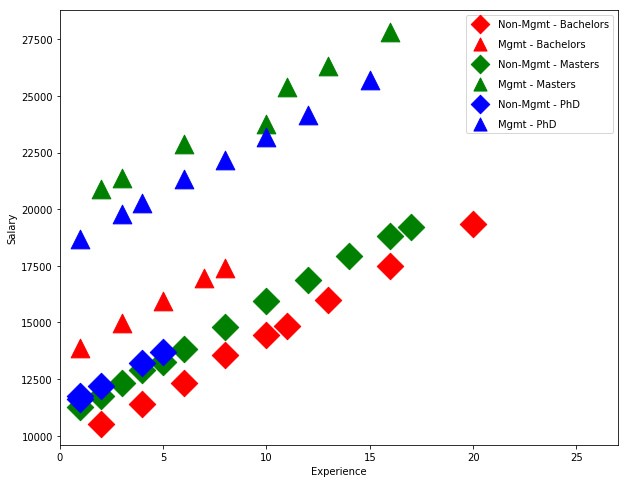### Define and Fit a Linear Model¶

Fit a linear model

$$S_i = \beta_0 + \beta_1X_i + \beta_2E_{i2} + \beta_3E_{i3} + \beta_4M_i + \epsilon_i$$

where

$$E_{i2}=\cases{1,&if E_i=2;\cr 0,&otherwise. \cr}$$

$$E_{i3}=\cases{1,&if E_i=3;\cr 0,&otherwise. \cr}$$

In the following, the model is defined using "patsy".

• An intercept is automatically included.
• C(variable) includes the variable automatically as categories.
In :
formula = 'S ~ C(E) + C(M) + X'
lm = ols(formula, salary_table).fit()
print(lm.summary())

                            OLS Regression Results
==============================================================================
Dep. Variable:                      S   R-squared:                       0.957
Method:                 Least Squares   F-statistic:                     226.8
Date:                Sat, 04 Feb 2017   Prob (F-statistic):           2.23e-27
Time:                        17:08:50   Log-Likelihood:                -381.63
No. Observations:                  46   AIC:                             773.3
Df Residuals:                      41   BIC:                             782.4
Df Model:                           4
Covariance Type:            nonrobust
==============================================================================
coef    std err          t      P>|t|      [0.025      0.975]
------------------------------------------------------------------------------
Intercept   8035.5976    386.689     20.781      0.000    7254.663    8816.532
C(E)[T.2]   3144.0352    361.968      8.686      0.000    2413.025    3875.045
C(E)[T.3]   2996.2103    411.753      7.277      0.000    2164.659    3827.762
C(M)[T.1]   6883.5310    313.919     21.928      0.000    6249.559    7517.503
X            546.1840     30.519     17.896      0.000     484.549     607.819
==============================================================================
Omnibus:                        2.293   Durbin-Watson:                   2.237
Prob(Omnibus):                  0.318   Jarque-Bera (JB):                1.362
Skew:                          -0.077   Prob(JB):                        0.506
Kurtosis:                       2.171   Cond. No.                         33.5
==============================================================================

Warnings:
 Standard Errors assume that the covariance matrix of the errors is correctly specified.


Dep. Variable the variable to be fitted; here, the "Salary"

Model OLS = ordinary least squares

Df Residuals The number of observations, minus the number of parameters fitted.

Df model "Degree of Freedom" of the model, i.e. the dimensionnality of the subspace spanned by the model. This entails that the intercept is not counted.

R-squared Coefficient of Determination = (S0-Sm)/S0

Adj. R-squared The adjusted R2 coefficient, which takes into consideration the number of model paramters.

F-statistic, and corresponding Prob F-test on the regression model, if it is significantly different from the minimum model (i.e. a constant offset only)

Log-Likelihood Maximum log-likelihood value for the model.

AIC Aiken's Information Criterion, for the assessment of the model.

BIC Bayesian Information Criterion, for the assessment of the model.

The values in the lowest box describe properties of the residuals ("Skew", "Kurtosisi"), as well as tests on the residuals.

### Inspect the Design Matrix, and demonstrate the Model Predictions¶

Look at the design matrix created for us. Every results instance has a reference to the model.

In :
lm.model.exog[:10]

Out:
array([[ 1.,  0.,  0.,  1.,  1.],
[ 1.,  0.,  1.,  0.,  1.],
[ 1.,  0.,  1.,  1.,  1.],
[ 1.,  1.,  0.,  0.,  1.],
[ 1.,  0.,  1.,  0.,  1.],
[ 1.,  1.,  0.,  1.,  2.],
[ 1.,  1.,  0.,  0.,  2.],
[ 1.,  0.,  0.,  0.,  2.],
[ 1.,  0.,  1.,  0.,  2.],
[ 1.,  1.,  0.,  0.,  3.]])

Since we initially passed in a DataFrame, we have a transformed DataFrame available.

In :
print(lm.model.data.orig_exog.head(10))

   Intercept  C(E)[T.2]  C(E)[T.3]  C(M)[T.1]    X
0        1.0        0.0        0.0        1.0  1.0
1        1.0        0.0        1.0        0.0  1.0
2        1.0        0.0        1.0        1.0  1.0
3        1.0        1.0        0.0        0.0  1.0
4        1.0        0.0        1.0        0.0  1.0
5        1.0        1.0        0.0        1.0  2.0
6        1.0        1.0        0.0        0.0  2.0
7        1.0        0.0        0.0        0.0  2.0
8        1.0        0.0        1.0        0.0  2.0
9        1.0        1.0        0.0        0.0  3.0


There is a reference to the original untouched data in

In :
print(lm.model.data.frame.head(10))

       S  X  E  M
0  13876  1  1  1
1  11608  1  3  0
2  18701  1  3  1
3  11283  1  2  0
4  11767  1  3  0
5  20872  2  2  1
6  11772  2  2  0
7  10535  2  1  0
8  12195  2  3  0
9  12313  3  2  0


If you use the formula interface, statsmodels remembers this transformation. Say you want to know the predicted salary for someone with 12 years experience and a Master's degree who is in a management position

In :
lm.predict(pd.DataFrame({'X' : , 'M' : , 'E' : }))

Out:
0    24617.372072
dtype: float64

### Check the Residuals¶

So far we've assumed that the effect of experience is the same for each level of education and professional role. Perhaps this assumption isn't merited. We can formally test this using some interactions.

We can start by seeing if our model assumptions are met. Let's look at a residuals plot, with the groups separated.

In :
resid = lm.resid

In :
fig = plt.figure(figsize=(12,8))
xticks = []
ax = fig.add_subplot(111, xlabel='Group (E, M)', ylabel='Residuals')
for values, group in factor_groups:
i,j = values
xticks.append(str((i, j)))
group_num = i*2 + j - 1 # for plotting purposes
x = [group_num] * len(group)
ax.scatter(x, resid[group.index], marker=symbols[j], color=colors[i-1],
s=144, edgecolors='black')
ax.set_xticks([1,2,3,4,5,6])
ax.set_xticklabels(xticks)
ax.axis('tight');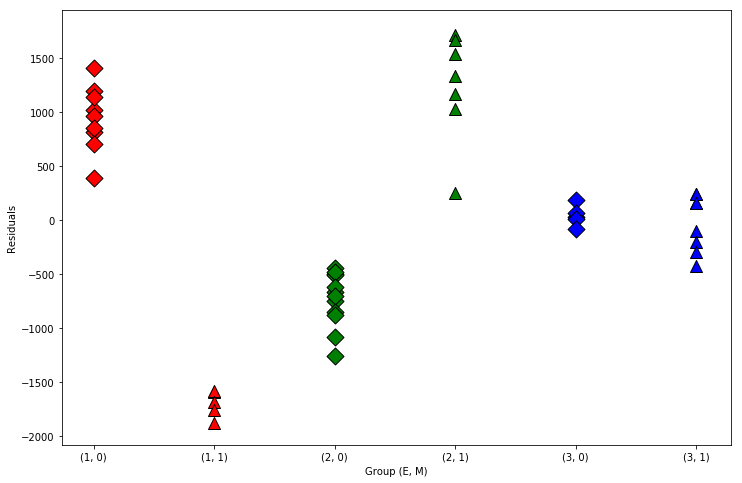Obviously, the linear model alone does not capture all model features, as the residuals are not normally distributed within each group. To improve the model, we check if interactions between the model parameters can explain the group differences.

#### Interaction Salary*Experience¶

Add an interaction between salary and experience, allowing different intercepts for level of experience.

$$S_i = \beta_0+\beta_1X_i+\beta_2E_{i2}+\beta_3E_{i3}+\beta_4M_i+\beta_5E_{i2}X_i+\beta_6E_{i3}X_i+\epsilon_i$$
In :
interX_lm = ols('S ~ C(E)*X + C(M)', salary_table).fit()
print(interX_lm.summary())

                            OLS Regression Results
==============================================================================
Dep. Variable:                      S   R-squared:                       0.961
Method:                 Least Squares   F-statistic:                     158.6
Date:                Sat, 04 Feb 2017   Prob (F-statistic):           8.23e-26
Time:                        17:09:30   Log-Likelihood:                -379.47
No. Observations:                  46   AIC:                             772.9
Df Residuals:                      39   BIC:                             785.7
Df Model:                           6
Covariance Type:            nonrobust
===============================================================================
coef    std err          t      P>|t|      [0.025      0.975]
-------------------------------------------------------------------------------
Intercept    7256.2800    549.494     13.205      0.000    6144.824    8367.736
C(E)[T.2]    4172.5045    674.966      6.182      0.000    2807.256    5537.753
C(E)[T.3]    3946.3649    686.693      5.747      0.000    2557.396    5335.333
C(M)[T.1]    7102.4539    333.442     21.300      0.000    6428.005    7776.903
X             632.2878     53.185     11.888      0.000     524.710     739.865
C(E)[T.2]:X  -125.5147     69.863     -1.797      0.080    -266.826      15.796
C(E)[T.3]:X  -141.2741     89.281     -1.582      0.122    -321.861      39.313
==============================================================================
Omnibus:                        0.432   Durbin-Watson:                   2.179
Prob(Omnibus):                  0.806   Jarque-Bera (JB):                0.590
Skew:                           0.144   Prob(JB):                        0.744
Kurtosis:                       2.526   Cond. No.                         69.7
==============================================================================

Warnings:
 Standard Errors assume that the covariance matrix of the errors is correctly specified.


The "Factor" Experience has the "Treatments" (or elements) "1", "2", and "3". Since the "Treatment 1"([T.1]) is taken as the reference, it is not listed here explicitly. This is called "corner-point" approach. Similarly, the "Factor" Management has the "Treatments" "0" and "1". With "0" taken as the reference, only the term C(M)[T.1] is listed in the model. The interactions are described by the terms "C(E)[T.i]:X".

##### Test the Interaction Management*Experience¶

Test that $\beta_5 = \beta_6 = 0$. We can use anova_lm or we can use an F-test.

In :
print(anova_lm(lm, interX_lm))

   df_resid           ssr  df_diff       ss_diff         F    Pr(>F)
0      41.0  4.328072e+07      0.0           NaN       NaN       NaN
1      39.0  3.941068e+07      2.0  3.870040e+06  1.914856  0.160964

C:\Programs\WinPython-64bit-3.6.0.1Qt5\python-3.6.0.amd64\lib\site-packages\scipy\stats\_distn_infrastructure.py:875: RuntimeWarning: invalid value encountered in greater
return (self.a < x) & (x < self.b)
C:\Programs\WinPython-64bit-3.6.0.1Qt5\python-3.6.0.amd64\lib\site-packages\scipy\stats\_distn_infrastructure.py:875: RuntimeWarning: invalid value encountered in less
return (self.a < x) & (x < self.b)
C:\Programs\WinPython-64bit-3.6.0.1Qt5\python-3.6.0.amd64\lib\site-packages\scipy\stats\_distn_infrastructure.py:1814: RuntimeWarning: invalid value encountered in less_equal
cond2 = cond0 & (x <= self.a)

In :
print(interX_lm.f_test('C(E)[T.2]:X = C(E)[T.3]:X = 0'))

<F test: F=array([[ 1.91485593]]), p=0.16096422424743717, df_denom=39, df_num=2>

In :
print(interX_lm.f_test([[0,0,0,0,0,1,-1],[0,0,0,0,0,0,1]]))

<F test: F=array([[ 1.91485593]]), p=0.16096422424743717, df_denom=39, df_num=2>


Both tests show that the models are not significantly different. In other words, there is no interaction effect between Management and Experience in the data.

The contrasts are created here under the hood by patsy.

Recall that F-tests are of the form $R\beta = q$

In :
LC = interX_lm.model.data.orig_exog.design_info.linear_constraint('C(E)[T.2]:X = C(E)[T.3]:X = 0')
print(LC.coefs)
print(LC.constants)

[[ 0.  0.  0.  0.  0.  1. -1.]
[ 0.  0.  0.  0.  0.  0.  1.]]
[[ 0.]
[ 0.]]


#### Interact education with management¶

In :
interM_lm = ols('S ~ X + C(E)*C(M)', salary_table).fit()
print(interM_lm.summary())

                            OLS Regression Results
==============================================================================
Dep. Variable:                      S   R-squared:                       0.999
Method:                 Least Squares   F-statistic:                     5517.
Date:                Sat, 04 Feb 2017   Prob (F-statistic):           1.67e-55
Time:                        17:09:31   Log-Likelihood:                -298.74
No. Observations:                  46   AIC:                             611.5
Df Residuals:                      39   BIC:                             624.3
Df Model:                           6
Covariance Type:            nonrobust
=======================================================================================
coef    std err          t      P>|t|      [0.025      0.975]
---------------------------------------------------------------------------------------
Intercept            9472.6854     80.344    117.902      0.000    9310.175    9635.196
C(E)[T.2]            1381.6706     77.319     17.870      0.000    1225.279    1538.063
C(E)[T.3]            1730.7483    105.334     16.431      0.000    1517.690    1943.806
C(M)[T.1]            3981.3769    101.175     39.351      0.000    3776.732    4186.022
C(E)[T.2]:C(M)[T.1]  4902.5231    131.359     37.322      0.000    4636.825    5168.222
C(E)[T.3]:C(M)[T.1]  3066.0351    149.330     20.532      0.000    2763.986    3368.084
X                     496.9870      5.566     89.283      0.000     485.728     508.246
==============================================================================
Omnibus:                       74.761   Durbin-Watson:                   2.244
Prob(Omnibus):                  0.000   Jarque-Bera (JB):             1037.873
Skew:                          -4.103   Prob(JB):                    4.25e-226
Kurtosis:                      24.776   Cond. No.                         79.0
==============================================================================

Warnings:
 Standard Errors assume that the covariance matrix of the errors is correctly specified.

In :
print(anova_lm(lm, interM_lm))

   df_resid           ssr  df_diff       ss_diff           F        Pr(>F)
0      41.0  4.328072e+07      0.0           NaN         NaN           NaN
1      39.0  1.178168e+06      2.0  4.210255e+07  696.844466  3.025504e-31

C:\Programs\WinPython-64bit-3.6.0.1Qt5\python-3.6.0.amd64\lib\site-packages\scipy\stats\_distn_infrastructure.py:875: RuntimeWarning: invalid value encountered in greater
return (self.a < x) & (x < self.b)
C:\Programs\WinPython-64bit-3.6.0.1Qt5\python-3.6.0.amd64\lib\site-packages\scipy\stats\_distn_infrastructure.py:875: RuntimeWarning: invalid value encountered in less
return (self.a < x) & (x < self.b)
C:\Programs\WinPython-64bit-3.6.0.1Qt5\python-3.6.0.amd64\lib\site-packages\scipy\stats\_distn_infrastructure.py:1814: RuntimeWarning: invalid value encountered in less_equal
cond2 = cond0 & (x <= self.a)

The interaction effect Management*Education is highly significant!
##### Check the residuals of the extended model¶
In :
infl = interM_lm.get_influence()
resid = infl.resid_studentized_internal

In :
fig = plt.figure(figsize=(12,8))
ax = fig.add_subplot(111, xlabel='X', ylabel='standardized resids')

for values, group in factor_groups:
i,j = values
idx = group.index
ax.scatter(X[idx], resid[idx], marker=symbols[j], color=colors[i-1],
s=144, edgecolors='black')
ax.axis('tight');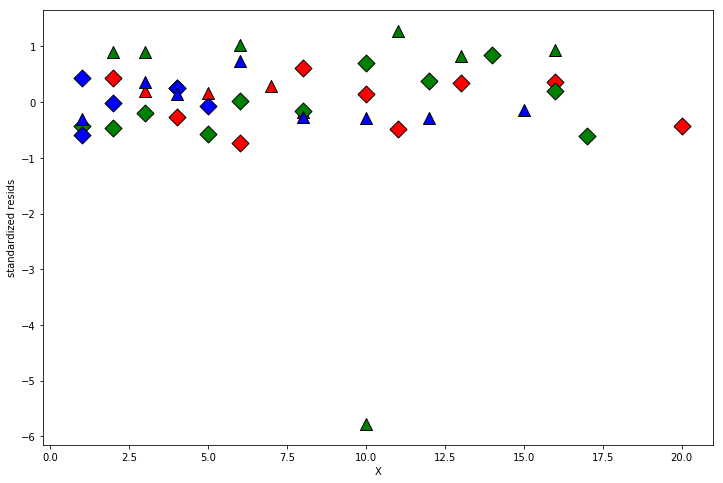There looks to be an outlier.

#### Find and Remove the Outlier¶

In :
outl = interM_lm.outlier_test('fdr_bh')
print(outl)

    student_resid       unadj_p     fdr_bh(p)
32     -14.950832  1.676948e-17  7.713960e-16
33       1.288001  2.055339e-01  9.599254e-01
23       1.023194  3.126860e-01  9.599254e-01
41       0.942329  3.519767e-01  9.599254e-01
11       0.896574  3.755915e-01  9.599254e-01
5        0.890643  3.787254e-01  9.599254e-01
39       0.835081  4.088920e-01  9.599254e-01
38       0.819164  4.178011e-01  9.599254e-01
21       0.729762  4.700104e-01  9.599254e-01
20      -0.726132  4.722071e-01  9.599254e-01
30       0.704477  4.854309e-01  9.599254e-01
28       0.603122  5.500109e-01  9.599254e-01
44      -0.599720  5.522526e-01  9.599254e-01
1       -0.590487  5.583601e-01  9.599254e-01
17      -0.564832  5.755078e-01  9.599254e-01
0       -0.482474  6.322368e-01  9.599254e-01
34      -0.474793  6.376517e-01  9.599254e-01
6       -0.464031  6.452728e-01  9.599254e-01
7        0.428795  6.704934e-01  9.599254e-01
45      -0.426443  6.721915e-01  9.599254e-01
4        0.424446  6.736338e-01  9.599254e-01
3       -0.418531  6.779148e-01  9.599254e-01
35       0.382327  7.043486e-01  9.599254e-01
43       0.360696  7.203240e-01  9.599254e-01
12       0.357944  7.223662e-01  9.599254e-01
37       0.342548  7.338259e-01  9.599254e-01
2       -0.291688  7.721113e-01  9.599254e-01
24       0.287114  7.755852e-01  9.599254e-01
31      -0.285085  7.771273e-01  9.599254e-01
36      -0.275068  7.847542e-01  9.599254e-01
13      -0.268726  7.895942e-01  9.599254e-01
27      -0.259790  7.964281e-01  9.599254e-01
15       0.252085  8.023339e-01  9.599254e-01
16       0.249972  8.039551e-01  9.599254e-01
42       0.195876  8.457513e-01  9.599254e-01
9       -0.194723  8.466475e-01  9.599254e-01
10       0.190826  8.496777e-01  9.599254e-01
26      -0.165977  8.690547e-01  9.599254e-01
19       0.165168  8.696870e-01  9.599254e-01
25      -0.161711  8.723902e-01  9.599254e-01
14       0.147997  8.831273e-01  9.599254e-01
29       0.147288  8.836831e-01  9.599254e-01
40      -0.129912  8.973215e-01  9.599254e-01
18      -0.072460  9.426159e-01  9.854621e-01
22       0.016188  9.871691e-01  9.878792e-01
8       -0.015292  9.878792e-01  9.878792e-01

C:\Programs\WinPython-64bit-3.6.0.1Qt5\python-3.6.0.amd64\lib\site-packages\ipykernel\__main__.py:2: FutureWarning: sort(columns=....) is deprecated, use sort_values(by=.....)
from ipykernel import kernelapp as app

In :
idx = salary_table.index.drop(32)

In :
print(idx)

Int64Index([ 0,  1,  2,  3,  4,  5,  6,  7,  8,  9, 10, 11, 12, 13, 14, 15, 16,
17, 18, 19, 20, 21, 22, 23, 24, 25, 26, 27, 28, 29, 30, 31, 33, 34,
35, 36, 37, 38, 39, 40, 41, 42, 43, 44, 45],
dtype='int64')

##### Rerun the original linear model without the outlier¶
In :
lm32 = ols('S ~ C(E) + X + C(M)', data=salary_table, subset=idx).fit()
print(lm32.summary())

                            OLS Regression Results
==============================================================================
Dep. Variable:                      S   R-squared:                       0.955
Method:                 Least Squares   F-statistic:                     211.7
Date:                Sat, 04 Feb 2017   Prob (F-statistic):           2.45e-26
Time:                        17:09:31   Log-Likelihood:                -373.79
No. Observations:                  45   AIC:                             757.6
Df Residuals:                      40   BIC:                             766.6
Df Model:                           4
Covariance Type:            nonrobust
==============================================================================
coef    std err          t      P>|t|      [0.025      0.975]
------------------------------------------------------------------------------
Intercept   8044.7518    392.781     20.482      0.000    7250.911    8838.592
C(E)[T.2]   3129.5286    370.470      8.447      0.000    2380.780    3878.277
C(E)[T.3]   2999.4451    416.712      7.198      0.000    2157.238    3841.652
C(M)[T.1]   6866.9856    323.991     21.195      0.000    6212.175    7521.796
X            545.7855     30.912     17.656      0.000     483.311     608.260
==============================================================================
Omnibus:                        2.511   Durbin-Watson:                   2.265
Prob(Omnibus):                  0.285   Jarque-Bera (JB):                1.400
Skew:                          -0.044   Prob(JB):                        0.496
Kurtosis:                       2.140   Cond. No.                         33.1
==============================================================================

Warnings:
 Standard Errors assume that the covariance matrix of the errors is correctly specified.


#### Interaction Education*Experience¶

In :
interX_lm32 = ols('S ~ C(E) * X + C(M)', data=salary_table, subset=idx).fit()
print(interX_lm32.summary())

                            OLS Regression Results
==============================================================================
Dep. Variable:                      S   R-squared:                       0.959
Method:                 Least Squares   F-statistic:                     147.7
Date:                Sat, 04 Feb 2017   Prob (F-statistic):           8.97e-25
Time:                        17:09:32   Log-Likelihood:                -371.70
No. Observations:                  45   AIC:                             757.4
Df Residuals:                      38   BIC:                             770.0
Df Model:                           6
Covariance Type:            nonrobust
===============================================================================
coef    std err          t      P>|t|      [0.025      0.975]
-------------------------------------------------------------------------------
Intercept    7266.0887    558.872     13.001      0.000    6134.711    8397.466
C(E)[T.2]    4162.0846    685.728      6.070      0.000    2773.900    5550.269
C(E)[T.3]    3940.4359    696.067      5.661      0.000    2531.322    5349.549
C(M)[T.1]    7088.6387    345.587     20.512      0.000    6389.035    7788.243
X             631.6892     53.950     11.709      0.000     522.473     740.905
C(E)[T.2]:X  -125.5009     70.744     -1.774      0.084    -268.714      17.712
C(E)[T.3]:X  -139.8410     90.728     -1.541      0.132    -323.511      43.829
==============================================================================
Omnibus:                        0.617   Durbin-Watson:                   2.194
Prob(Omnibus):                  0.734   Jarque-Bera (JB):                0.728
Skew:                           0.162   Prob(JB):                        0.695
Kurtosis:                       2.468   Cond. No.                         68.7
==============================================================================

Warnings:
 Standard Errors assume that the covariance matrix of the errors is correctly specified.

In :
table3 = anova_lm(lm32, interX_lm32)
print(table3)

   df_resid           ssr  df_diff       ss_diff         F    Pr(>F)
0      40.0  4.320910e+07      0.0           NaN       NaN       NaN
1      38.0  3.937424e+07      2.0  3.834859e+06  1.850508  0.171042

C:\Programs\WinPython-64bit-3.6.0.1Qt5\python-3.6.0.amd64\lib\site-packages\scipy\stats\_distn_infrastructure.py:875: RuntimeWarning: invalid value encountered in greater
return (self.a < x) & (x < self.b)
C:\Programs\WinPython-64bit-3.6.0.1Qt5\python-3.6.0.amd64\lib\site-packages\scipy\stats\_distn_infrastructure.py:875: RuntimeWarning: invalid value encountered in less
return (self.a < x) & (x < self.b)
C:\Programs\WinPython-64bit-3.6.0.1Qt5\python-3.6.0.amd64\lib\site-packages\scipy\stats\_distn_infrastructure.py:1814: RuntimeWarning: invalid value encountered in less_equal
cond2 = cond0 & (x <= self.a)


Again, this is not significant, and can be left away.

### Final Result¶

Result from the Interaction Test: the significant model with Interaction Experience*Management, without the outlier #32

In :
interM_lm32 = ols('S ~ X + C(E) * C(M)', data=salary_table, subset=idx).fit()
print(anova_lm(lm32, interM_lm32))

   df_resid           ssr  df_diff       ss_diff            F        Pr(>F)
0      40.0  4.320910e+07      0.0           NaN          NaN           NaN
1      38.0  1.711881e+05      2.0  4.303791e+07  4776.734853  2.291239e-46

C:\Programs\WinPython-64bit-3.6.0.1Qt5\python-3.6.0.amd64\lib\site-packages\scipy\stats\_distn_infrastructure.py:875: RuntimeWarning: invalid value encountered in greater
return (self.a < x) & (x < self.b)
C:\Programs\WinPython-64bit-3.6.0.1Qt5\python-3.6.0.amd64\lib\site-packages\scipy\stats\_distn_infrastructure.py:875: RuntimeWarning: invalid value encountered in less
return (self.a < x) & (x < self.b)
C:\Programs\WinPython-64bit-3.6.0.1Qt5\python-3.6.0.amd64\lib\site-packages\scipy\stats\_distn_infrastructure.py:1814: RuntimeWarning: invalid value encountered in less_equal
cond2 = cond0 & (x <= self.a)


Re-plotting the residuals

In :
resid = interM_lm32.get_influence().summary_frame()['standard_resid']
fig = plt.figure(figsize=(12,8))
ax = fig.add_subplot(111, xlabel='X[~]', ylabel='standardized resids')

for values, group in factor_groups:
i,j = values
idx = group.index
ax.scatter(X[idx], resid[idx], marker=symbols[j], color=colors[i-1],
s=144, edgecolors='black')
ax.axis('tight');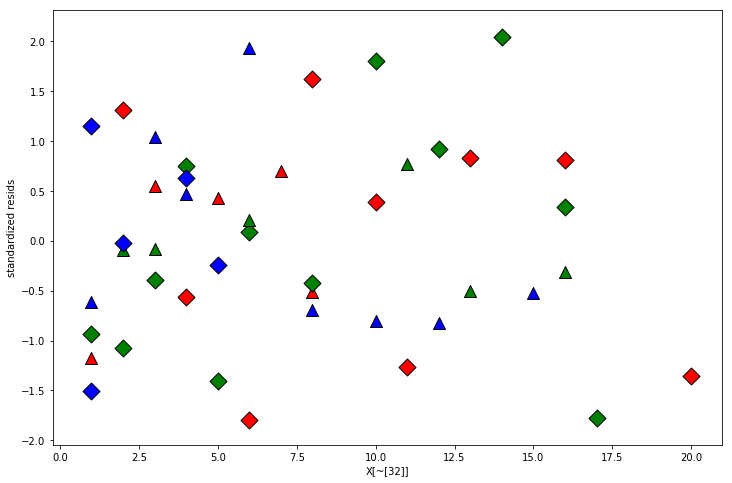A final plot of the fitted values

In :
lm_final = ols('S ~ X + C(E)*C(M)', data=salary_table.drop()).fit()
mf = lm_final.model.data.orig_exog
lstyle = ['-','--']

fig = plt.figure(figsize=(12,8))

for values, group in factor_groups:
i,j = values
idx = group.index
ax.scatter(X[idx], S[idx], marker=symbols[j], color=colors[i-1],
s=144, edgecolors='black')
# drop NA because there is no idx 32 in the final model
ax.plot(mf.X[idx].dropna(), lm_final.fittedvalues[idx].dropna(),
ls=lstyle[j], color=colors[i-1])
ax.axis('tight');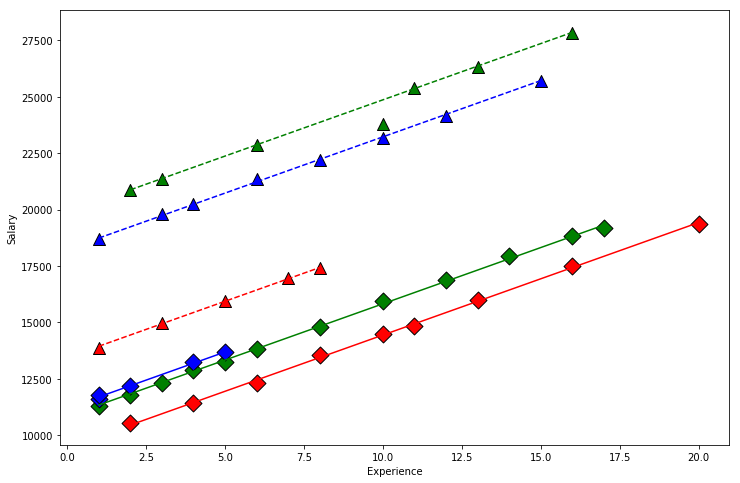##### Interaction Plot Salary | Experience¶

From our first look at the data, the difference between Master's and PhD in the management group is different than in the non-management group. This is an interaction between the two qualitative variables management, M and education, E. We can visualize this by first removing the effect of experience, then plotting the means within each of the 6 groups using interaction.plot.

In :
U = S - X * interX_lm32.params['X']
U.name = 'Salary|X'

fig = plt.figure(figsize=(12,8))
ax = interaction_plot(E, M, U, colors=['red','blue'], markers=['^','D'],
markersize=10, ax=ax)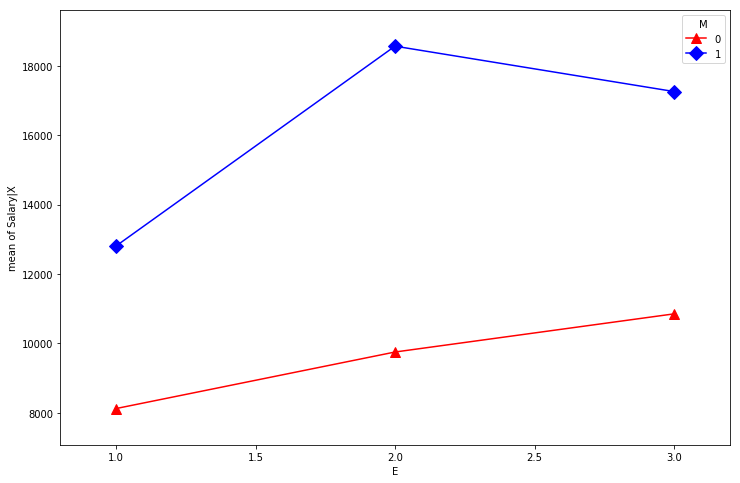## Example 2: Minority Employment Data - ABLine plotting¶

TEST - Job Aptitude Test Score ETHN - 1 if minority, 0 otherwise JPERF - Job performance evaluation
In :
from urllib.request import urlopen
url_base = 'https://raw.githubusercontent.com/thomas-haslwanter/statsintro_python/master/ipynb/Data/data_others/'
inFile = 'minority.table'
url = url_base + inFile

minority_table.to_csv('minority.table', sep="\t", index=False)

In :
factor_group = minority_table.groupby(['ETHN'])

fig = plt.figure(figsize=(12,8))
colors = ['purple', 'green']
markers = ['o', 'v']
for factor, group in factor_group:
ax.scatter(group['TEST'], group['JPERF'], color=colors[factor],
marker=markers[factor], s=12**2)
ax.legend(['ETHN == 1', 'ETHN == 0'], scatterpoints=1)

Out:
<matplotlib.legend.Legend at 0x2d8af7ccfd0>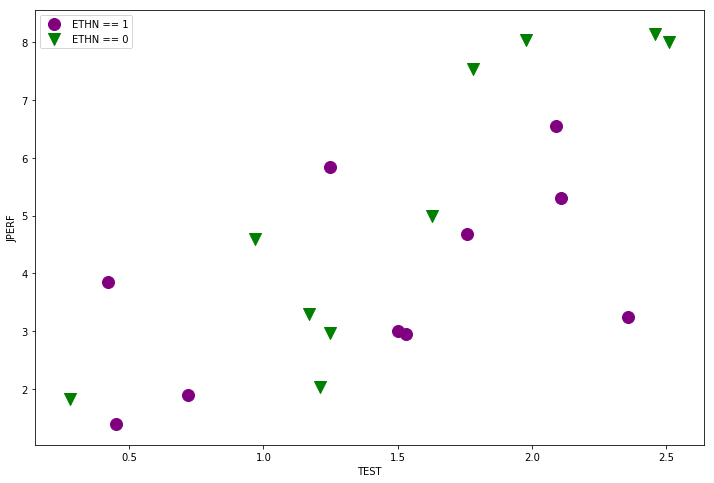In :
min_lm = ols('JPERF ~ TEST', data=minority_table).fit()
print(min_lm.summary())

                            OLS Regression Results
==============================================================================
Dep. Variable:                  JPERF   R-squared:                       0.517
Method:                 Least Squares   F-statistic:                     19.25
Date:                Sat, 04 Feb 2017   Prob (F-statistic):           0.000356
Time:                        17:09:34   Log-Likelihood:                -36.614
No. Observations:                  20   AIC:                             77.23
Df Residuals:                      18   BIC:                             79.22
Df Model:                           1
Covariance Type:            nonrobust
==============================================================================
coef    std err          t      P>|t|      [0.025      0.975]
------------------------------------------------------------------------------
Intercept      1.0350      0.868      1.192      0.249      -0.789       2.859
TEST           2.3605      0.538      4.387      0.000       1.230       3.491
==============================================================================
Omnibus:                        0.324   Durbin-Watson:                   2.896
Prob(Omnibus):                  0.850   Jarque-Bera (JB):                0.483
Skew:                          -0.186   Prob(JB):                        0.785
Kurtosis:                       2.336   Cond. No.                         5.26
==============================================================================

Warnings:
 Standard Errors assume that the covariance matrix of the errors is correctly specified.

In :
fig = plt.figure(figsize=(12,8))
for factor, group in factor_group:
ax.scatter(group['TEST'], group['JPERF'], color=colors[factor],
marker=markers[factor], s=12**2)
ax.legend(['ETHN == 1', 'ETHN == 0'], scatterpoints=1, loc='upper left')
fig = abline_plot(model_results = min_lm, ax=ax)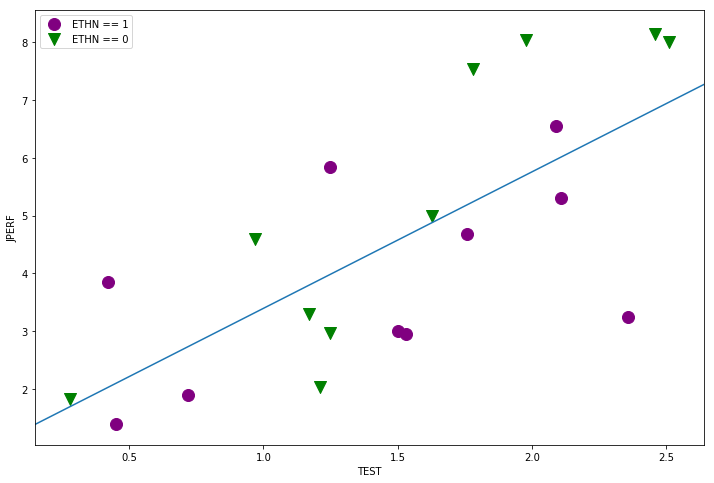In :
min_lm2 = ols('JPERF ~ TEST + TEST:ETHN', data=minority_table).fit()
print(min_lm2.summary())

                            OLS Regression Results
==============================================================================
Dep. Variable:                  JPERF   R-squared:                       0.632
Method:                 Least Squares   F-statistic:                     14.59
Date:                Sat, 04 Feb 2017   Prob (F-statistic):           0.000204
Time:                        17:09:35   Log-Likelihood:                -33.891
No. Observations:                  20   AIC:                             73.78
Df Residuals:                      17   BIC:                             76.77
Df Model:                           2
Covariance Type:            nonrobust
==============================================================================
coef    std err          t      P>|t|      [0.025      0.975]
------------------------------------------------------------------------------
Intercept      1.1211      0.780      1.437      0.169      -0.525       2.768
TEST           1.8276      0.536      3.412      0.003       0.698       2.958
TEST:ETHN      0.9161      0.397      2.306      0.034       0.078       1.754
==============================================================================
Omnibus:                        0.388   Durbin-Watson:                   3.008
Prob(Omnibus):                  0.823   Jarque-Bera (JB):                0.514
Skew:                           0.050   Prob(JB):                        0.773
Kurtosis:                       2.221   Cond. No.                         5.96
==============================================================================

Warnings:
 Standard Errors assume that the covariance matrix of the errors is correctly specified.

In :
fig = plt.figure(figsize=(12,8))
for factor, group in factor_group:
ax.scatter(group['TEST'], group['JPERF'], color=colors[factor],
marker=markers[factor], s=12**2)

fig = abline_plot(intercept = min_lm2.params['Intercept'],
slope = min_lm2.params['TEST'], ax=ax, color='purple')
ax = fig.axes
fig = abline_plot(intercept = min_lm2.params['Intercept'],
slope = min_lm2.params['TEST'] + min_lm2.params['TEST:ETHN'],
ax=ax, color='green')
ax.legend(['ETHN == 1', 'ETHN == 0'], scatterpoints=1, loc='upper left');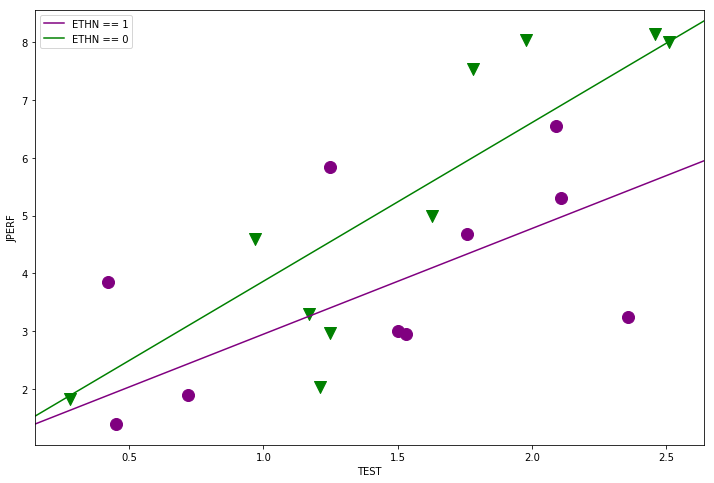In :
min_lm3 = ols('JPERF ~ TEST + ETHN', data=minority_table).fit()
print(min_lm3.summary())

                            OLS Regression Results
==============================================================================
Dep. Variable:                  JPERF   R-squared:                       0.572
Method:                 Least Squares   F-statistic:                     11.38
Date:                Sat, 04 Feb 2017   Prob (F-statistic):           0.000731
Time:                        17:09:35   Log-Likelihood:                -35.390
No. Observations:                  20   AIC:                             76.78
Df Residuals:                      17   BIC:                             79.77
Df Model:                           2
Covariance Type:            nonrobust
==============================================================================
coef    std err          t      P>|t|      [0.025      0.975]
------------------------------------------------------------------------------
Intercept      0.6120      0.887      0.690      0.500      -1.260       2.483
TEST           2.2988      0.522      4.400      0.000       1.197       3.401
ETHN           1.0276      0.691      1.487      0.155      -0.430       2.485
==============================================================================
Omnibus:                        0.251   Durbin-Watson:                   3.028
Prob(Omnibus):                  0.882   Jarque-Bera (JB):                0.437
Skew:                          -0.059   Prob(JB):                        0.804
Kurtosis:                       2.286   Cond. No.                         5.72
==============================================================================

Warnings:
 Standard Errors assume that the covariance matrix of the errors is correctly specified.

In :
fig = plt.figure(figsize=(12,8))
for factor, group in factor_group:
ax.scatter(group['TEST'], group['JPERF'], color=colors[factor],
marker=markers[factor], s=12**2)

fig = abline_plot(intercept = min_lm3.params['Intercept'],
slope = min_lm3.params['TEST'], ax=ax, color='purple')

ax = fig.axes
fig = abline_plot(intercept = min_lm3.params['Intercept'] + min_lm3.params['ETHN'],
slope = min_lm3.params['TEST'], ax=ax, color='green')
ax.legend(['ETHN == 1', 'ETHN == 0'], scatterpoints=1, loc='upper left');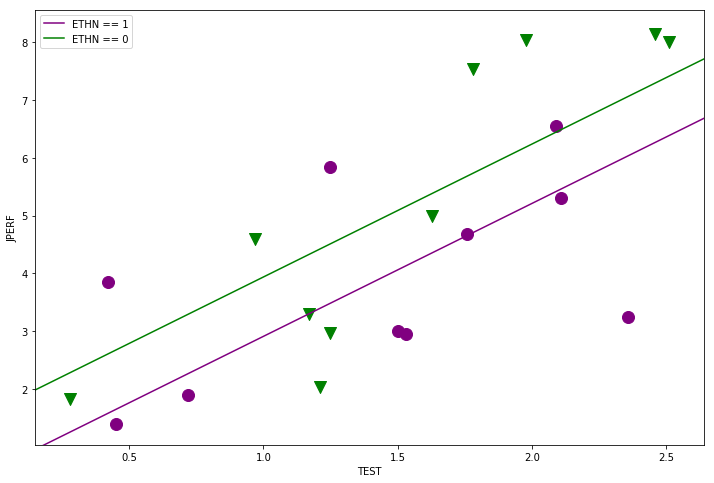In :
min_lm4 = ols('JPERF ~ TEST * ETHN', data=minority_table).fit()
print(min_lm4.summary())

                            OLS Regression Results
==============================================================================
Dep. Variable:                  JPERF   R-squared:                       0.664
Method:                 Least Squares   F-statistic:                     10.55
Date:                Sat, 04 Feb 2017   Prob (F-statistic):           0.000451
Time:                        17:09:35   Log-Likelihood:                -32.971
No. Observations:                  20   AIC:                             73.94
Df Residuals:                      16   BIC:                             77.92
Df Model:                           3
Covariance Type:            nonrobust
==============================================================================
coef    std err          t      P>|t|      [0.025      0.975]
------------------------------------------------------------------------------
Intercept      2.0103      1.050      1.914      0.074      -0.216       4.236
TEST           1.3134      0.670      1.959      0.068      -0.108       2.735
ETHN          -1.9132      1.540     -1.242      0.232      -5.179       1.352
TEST:ETHN      1.9975      0.954      2.093      0.053      -0.026       4.021
==============================================================================
Omnibus:                        3.377   Durbin-Watson:                   3.015
Prob(Omnibus):                  0.185   Jarque-Bera (JB):                1.330
Skew:                           0.120   Prob(JB):                        0.514
Kurtosis:                       1.760   Cond. No.                         13.8
==============================================================================

Warnings:
 Standard Errors assume that the covariance matrix of the errors is correctly specified.

In :
fig = plt.figure(figsize=(12,8))
for factor, group in factor_group:
ax.scatter(group['TEST'], group['JPERF'], color=colors[factor],
marker=markers[factor], s=12**2)

fig = abline_plot(intercept = min_lm4.params['Intercept'],
slope = min_lm4.params['TEST'], ax=ax, color='purple')
ax = fig.axes
fig = abline_plot(intercept = min_lm4.params['Intercept'] + min_lm4.params['ETHN'],
slope = min_lm4.params['TEST'] + min_lm4.params['TEST:ETHN'],
ax=ax, color='green')
ax.legend(['ETHN == 1', 'ETHN == 0'], scatterpoints=1, loc='upper left');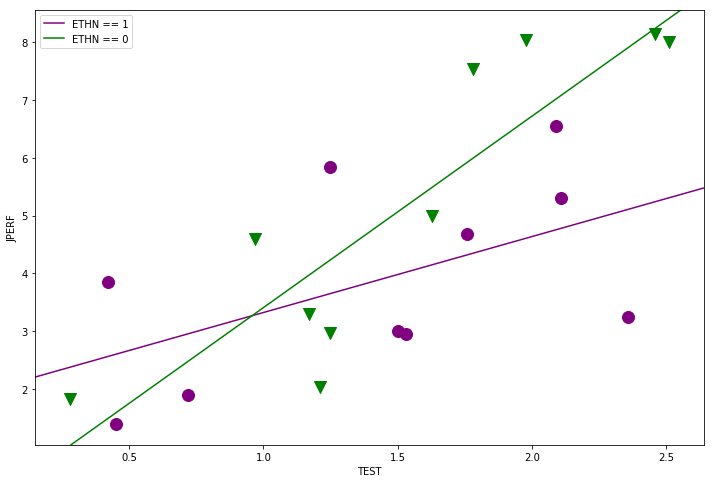Is there any effect of ETHN on slope or intercept?
Y ~ TEST vs. Y ~ TEST + ETHN + ETHN:TEST

In :
table5 = anova_lm(min_lm, min_lm4)
print(table5)

   df_resid        ssr  df_diff    ss_diff         F    Pr(>F)
0      18.0  45.568297      0.0        NaN       NaN       NaN
1      16.0  31.655473      2.0  13.912824  3.516061  0.054236

C:\Programs\WinPython-64bit-3.6.0.1Qt5\python-3.6.0.amd64\lib\site-packages\scipy\stats\_distn_infrastructure.py:875: RuntimeWarning: invalid value encountered in greater
return (self.a < x) & (x < self.b)
C:\Programs\WinPython-64bit-3.6.0.1Qt5\python-3.6.0.amd64\lib\site-packages\scipy\stats\_distn_infrastructure.py:875: RuntimeWarning: invalid value encountered in less
return (self.a < x) & (x < self.b)
C:\Programs\WinPython-64bit-3.6.0.1Qt5\python-3.6.0.amd64\lib\site-packages\scipy\stats\_distn_infrastructure.py:1814: RuntimeWarning: invalid value encountered in less_equal
cond2 = cond0 & (x <= self.a)


Is there any effect of ETHN on intercept?
Y ~ TEST vs. Y ~ TEST + ETHN

In :
table6 = anova_lm(min_lm, min_lm3)
print(table6)

   df_resid        ssr  df_diff   ss_diff         F    Pr(>F)
0      18.0  45.568297      0.0       NaN       NaN       NaN
1      17.0  40.321546      1.0  5.246751  2.212087  0.155246

C:\Programs\WinPython-64bit-3.6.0.1Qt5\python-3.6.0.amd64\lib\site-packages\scipy\stats\_distn_infrastructure.py:875: RuntimeWarning: invalid value encountered in greater
return (self.a < x) & (x < self.b)
C:\Programs\WinPython-64bit-3.6.0.1Qt5\python-3.6.0.amd64\lib\site-packages\scipy\stats\_distn_infrastructure.py:875: RuntimeWarning: invalid value encountered in less
return (self.a < x) & (x < self.b)
C:\Programs\WinPython-64bit-3.6.0.1Qt5\python-3.6.0.amd64\lib\site-packages\scipy\stats\_distn_infrastructure.py:1814: RuntimeWarning: invalid value encountered in less_equal
cond2 = cond0 & (x <= self.a)


Is there any effect of ETHN on slope?
Y ~ TEST vs. Y ~ TEST + ETHN:TEST

In :
table7 = anova_lm(min_lm, min_lm2)
print(table7)

   df_resid        ssr  df_diff    ss_diff         F    Pr(>F)
0      18.0  45.568297      0.0        NaN       NaN       NaN
1      17.0  34.707653      1.0  10.860644  5.319603  0.033949

C:\Programs\WinPython-64bit-3.6.0.1Qt5\python-3.6.0.amd64\lib\site-packages\scipy\stats\_distn_infrastructure.py:875: RuntimeWarning: invalid value encountered in greater
return (self.a < x) & (x < self.b)
C:\Programs\WinPython-64bit-3.6.0.1Qt5\python-3.6.0.amd64\lib\site-packages\scipy\stats\_distn_infrastructure.py:875: RuntimeWarning: invalid value encountered in less
return (self.a < x) & (x < self.b)
C:\Programs\WinPython-64bit-3.6.0.1Qt5\python-3.6.0.amd64\lib\site-packages\scipy\stats\_distn_infrastructure.py:1814: RuntimeWarning: invalid value encountered in less_equal
cond2 = cond0 & (x <= self.a)


Is it just the slope or both?
Y ~ TEST + ETHN:TEST vs Y ~ TEST + ETHN + ETHN:TEST

In :
table8 = anova_lm(min_lm2, min_lm4)
print(table8)

   df_resid        ssr  df_diff  ss_diff         F    Pr(>F)
0      17.0  34.707653      0.0      NaN       NaN       NaN
1      16.0  31.655473      1.0  3.05218  1.542699  0.232115

C:\Programs\WinPython-64bit-3.6.0.1Qt5\python-3.6.0.amd64\lib\site-packages\scipy\stats\_distn_infrastructure.py:875: RuntimeWarning: invalid value encountered in greater
return (self.a < x) & (x < self.b)
C:\Programs\WinPython-64bit-3.6.0.1Qt5\python-3.6.0.amd64\lib\site-packages\scipy\stats\_distn_infrastructure.py:875: RuntimeWarning: invalid value encountered in less
return (self.a < x) & (x < self.b)
C:\Programs\WinPython-64bit-3.6.0.1Qt5\python-3.6.0.amd64\lib\site-packages\scipy\stats\_distn_infrastructure.py:1814: RuntimeWarning: invalid value encountered in less_equal
cond2 = cond0 & (x <= self.a)


## Two Way ANOVA - Kidney failure data¶

Weight - (1,2,3) - Level of weight gan between treatments Duration - (1,2) - Level of duration of treatment Days - Time of stay in hospital
In :
try:
except:
url = 'http://stats191.stanford.edu/data/kidney.table'
kidney_table.to_csv("kidney.table", sep="\t", index=False)

C:\Programs\WinPython-64bit-3.6.0.1Qt5\python-3.6.0.amd64\lib\site-packages\ipykernel\__main__.py:5: ParserWarning: Falling back to the 'python' engine because the 'c' engine does not support regex separators (separators > 1 char and different from '\s+' are interpreted as regex); you can avoid this warning by specifying engine='python'.
C:\Programs\WinPython-64bit-3.6.0.1Qt5\python-3.6.0.amd64\lib\site-packages\pandas\io\parsers.py:1961: FutureWarning: split() requires a non-empty pattern match.
yield pat.split(line.strip())
C:\Programs\WinPython-64bit-3.6.0.1Qt5\python-3.6.0.amd64\lib\site-packages\pandas\io\parsers.py:1963: FutureWarning: split() requires a non-empty pattern match.
yield pat.split(line.strip())

In :
# Explore the dataset, it's a balanced design
print(kidney_table.groupby(['Weight', 'Duration']).size())

Weight  Duration
1       1           10
2           10
2       1           10
2           10
3       1           10
2           10
dtype: int64

In :
kt = kidney_table
fig = plt.figure(figsize=(10,8))
fig = interaction_plot(kt['Weight'], kt['Duration'], np.log(kt['Days']+1),
colors=['red', 'blue'], markers=['D','^'], ms=10, ax=ax)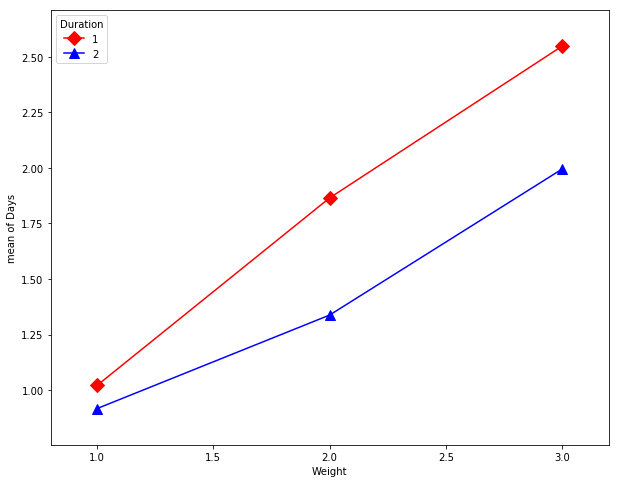$$Y_{ijk} = \mu + \alpha_i + \beta_j + \left(\alpha\beta\right)_{ij}+\epsilon_{ijk}$$

with

$$\epsilon_{ijk}\sim N\left(0,\sigma^2\right)$$
In :
help(anova_lm)

Help on function anova_lm in module statsmodels.stats.anova:

anova_lm(*args, **kwargs)
ANOVA table for one or more fitted linear models.

Parameters
----------
args : fitted linear model results instance
One or more fitted linear models
scale : float
Estimate of variance, If None, will be estimated from the largest
model. Default is None.
test : str {"F", "Chisq", "Cp"} or None
Test statistics to provide. Default is "F".
typ : str or int {"I","II","III"} or {1,2,3}
The type of ANOVA test to perform. See notes.
robust : {None, "hc0", "hc1", "hc2", "hc3"}
Use heteroscedasticity-corrected coefficient covariance matrix.
If robust covariance is desired, it is recommended to use hc3.

Returns
-------
anova : DataFrame
A DataFrame containing.

Notes
-----
Model statistics are given in the order of args. Models must have
been fit using the formula api.

--------
model_results.compare_f_test, model_results.compare_lm_test

Examples
--------
>>> import statsmodels.api as sm
>>> from statsmodels.formula.api import ols
>>> moore = sm.datasets.get_rdataset("Moore", "car", cache=True) # load
>>> data = moore.data
>>> data = data.rename(columns={"partner.status" :
...                             "partner_status"}) # make name pythonic
>>> moore_lm = ols('conformity ~ C(fcategory, Sum)*C(partner_status, Sum)',
...                 data=data).fit()
>>> table = sm.stats.anova_lm(moore_lm, typ=2) # Type 2 ANOVA DataFrame
>>> print(table)



Things available in the calling namespace are available in the formula evaluation namespace

In :
kidney_lm = ols('np.log(Days+1) ~ C(Duration) * C(Weight)', data=kt).fit()


ANOVA Type-I Sum of Squares

SS(A) for factor A.
SS(B|A) for factor B.
SS(AB|B, A) for interaction AB.

In :
print(anova_lm(kidney_lm))

                         df     sum_sq   mean_sq          F    PR(>F)
C(Duration)             1.0   2.339693  2.339693   4.358293  0.041562
C(Weight)               2.0  16.971291  8.485645  15.806745  0.000004
C(Duration):C(Weight)   2.0   0.635658  0.317829   0.592040  0.556748
Residual               54.0  28.989198  0.536837        NaN       NaN

C:\Programs\WinPython-64bit-3.6.0.1Qt5\python-3.6.0.amd64\lib\site-packages\scipy\stats\_distn_infrastructure.py:875: RuntimeWarning: invalid value encountered in greater
return (self.a < x) & (x < self.b)
C:\Programs\WinPython-64bit-3.6.0.1Qt5\python-3.6.0.amd64\lib\site-packages\scipy\stats\_distn_infrastructure.py:875: RuntimeWarning: invalid value encountered in less
return (self.a < x) & (x < self.b)
C:\Programs\WinPython-64bit-3.6.0.1Qt5\python-3.6.0.amd64\lib\site-packages\scipy\stats\_distn_infrastructure.py:1814: RuntimeWarning: invalid value encountered in less_equal
cond2 = cond0 & (x <= self.a)


ANOVA Type-II Sum of Squares

SS(A|B) for factor A.
SS(B|A) for factor B.

In :
print(anova_lm(kidney_lm, typ=2))

                          sum_sq    df          F    PR(>F)
C(Duration)             2.339693   1.0   4.358293  0.041562
C(Weight)              16.971291   2.0  15.806745  0.000004
C(Duration):C(Weight)   0.635658   2.0   0.592040  0.556748
Residual               28.989198  54.0        NaN       NaN

ANOVA Type-III Sum of Squares

SS(A|B, AB) for factor A.
SS(B|A, AB) for factor B.
In :
print(anova_lm(ols('np.log(Days+1) ~ C(Duration, Sum) * C(Weight, Poly)',
data=kt).fit(), typ=3))

                                      sum_sq    df           F        PR(>F)
Intercept                         156.301830   1.0  291.153237  2.077589e-23
C(Duration, Sum)                    2.339693   1.0    4.358293  4.156170e-02
C(Weight, Poly)                    16.971291   2.0   15.806745  3.944502e-06
C(Duration, Sum):C(Weight, Poly)    0.635658   2.0    0.592040  5.567479e-01
Residual                           28.989198  54.0         NaN           NaN